# HTML Tables

In this article, we will know the HTML Table, various ways to implement it, & will also understand its usage through the examples. HTML Table is an arrangement of data in rows and columns, or possibly in a more complex structure. Tables are widely used in communication, research, and data analysis. Tables are useful for various tasks such as presenting text information and numerical data. It can be used to compare two or more items in the tabular form layout. Tables are used to create databases.

Defining Tables in HTML: An HTML table is defined with the “table” tag. Each table row is defined with the “tr” tag. A table header is defined with the “th” tag. By default, table headings are bold and centered. A table data/cell is defined with the “td” tag.

Example 1: In this example, we are creating a simple table in HTML using a table tag.

## HTML

 `` `<``html``>`   `<``body``>` `    ``<``table``>` `        ``<``tr``>` `            ``<``th``>Book Name` `            ``<``th``>Author Name` `            ``<``th``>Genre` `        ``` `        ``<``tr``>` `            ``<``td``>The Book Thief` `            ``<``td``>Markus Zusak` `            ``<``td``>Historical Fiction` `        ``` `        ``<``tr``>` `            ``<``td``>The Cruel Prince` `            ``<``td``>Holly Black` `            ``<``td``>Fantasy` `        ``` `        ``<``tr``>` `            ``<``td``>The Silent Patient` `            ``<``td``> Alex Michaelides` `            ``<``td``>Psychological Fiction` `        ``` `    ``` ``   ``

Output: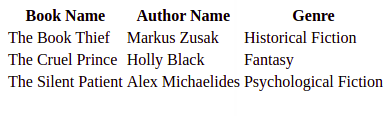HTML Table

Example 2: This example explains the use of the HTML Table.

## HTML

 `` `<``html``>`   `<``body``>` `    ``<``table``>` `        ``<``tr``>` `            ``<``th``>Firstname` `            ``<``th``>Lastname` `            ``<``th``>Age` `        ``` `        ``<``tr``>` `            ``<``td``>Priya` `            ``<``td``>Sharma` `            ``<``td``>24` `        ``` `        ``<``tr``>` `            ``<``td``>Arun` `            ``<``td``>Singh` `            ``<``td``>32` `        ``` `        ``<``tr``>` `            ``<``td``>Sam` `            ``<``td``>Watson` `            ``<``td``>41` `        ``` `    ``` ``   ``

Output: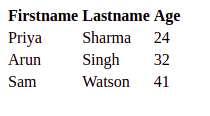Simple HTML Table

Accepted Attributes:

Adding a border to an HTML Table: A border is set using the CSS border property. If you do not specify a border for the table, it will be displayed without borders.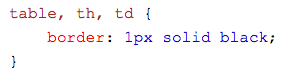Example 3: This example explains the addition of the border to the HTML Table.

## HTML

 `` `<``html``>`   `<``head``>` `    ``<``style``>` `    ``table,` `    ``th,` `    ``td {` `        ``border: 1px solid black;` `    ``}` `    ``` ``   `<``body``>` `    ``<``table` `style``=``"width:100%"``>` `        ``<``tr``>` `            ``<``th``>Firstname` `            ``<``th``>Lastname` `            ``<``th``>Age` `        ``` `        ``<``tr``>` `            ``<``td``>Priya` `            ``<``td``>Sharma` `            ``<``td``>24` `        ``` `        ``<``tr``>` `            ``<``td``>Arun` `            ``<``td``>Singh` `            ``<``td``>32` `        ``` `        ``<``tr``>` `            ``<``td``>Sam` `            ``<``td``>Watson` `            ``<``td``>41` `        ``` `    ``` ``   ``

Output: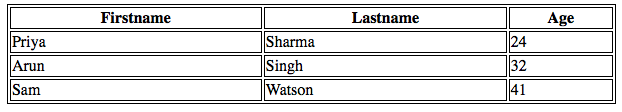HTML Table with border

Adding Collapsed Borders in an HTML Table: For borders to collapse into one border, add the CSS border-collapse property.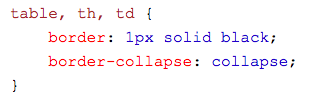Example 4: This example describes the addition of Collapsed Borders in HTML.

## HTML

 `` `<``html``>`   `<``head``>` `    ``<``style``>` `    ``table,` `    ``th,` `    ``td {` `        ``border: 1px solid black;` `        ``border-collapse: collapse;` `    ``}` `    ``` ``   `<``body``>` `    ``<``table` `style``=``"width:100%"``>` `        ``<``tr``>` `            ``<``th``>Firstname` `            ``<``th``>Lastname` `            ``<``th``>Age` `        ``` `        ``<``tr``>` `            ``<``td``>Priya` `            ``<``td``>Sharma` `            ``<``td``>24` `        ``` `        ``<``tr``>` `            ``<``td``>Arun` `            ``<``td``>Singh` `            ``<``td``>32` `        ``` `        ``<``tr``>` `            ``<``td``>Sam` `            ``<``td``>Watson` `            ``<``td``>41` `        ``` `    ``` ``   ``

Output: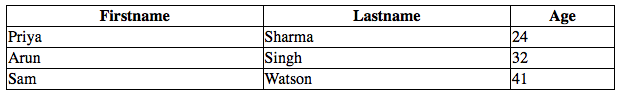HTML Table with Collapsed Borders

Adding Cell Padding in an HTML Table: Cell padding specifies the space between the cell content and its borders. If we do not specify a padding, the table cells will be displayed without padding.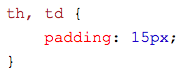Example 5: This example describes the addition of Table cell padding in HTML.

## HTML

 `` `<``html``>`   `<``head``>` `    ``<``style``>` `    ``table,` `    ``th,` `    ``td {` `        ``border: 1px solid black;` `        ``border-collapse: collapse;` `    ``}` `    `  `    ``th,` `    ``td {` `        ``padding: 20px;` `    ``}` `    ``` ``   `<``body``>` `    ``<``table` `style``=``"width:100%"``>` `        ``<``tr``>` `            ``<``th``>Firstname` `            ``<``th``>Lastname` `            ``<``th``>Age` `        ``` `        ``<``tr``>` `            ``<``td``>Priya` `            ``<``td``>Sharma` `            ``<``td``>24` `        ``` `        ``<``tr``>` `            ``<``td``>Arun` `            ``<``td``>Singh` `            ``<``td``>32` `        ``` `        ``<``tr``>` `            ``<``td``>Sam` `            ``<``td``>Watson` `            ``<``td``>41` `        ``` `    ``` ``   ``

Output: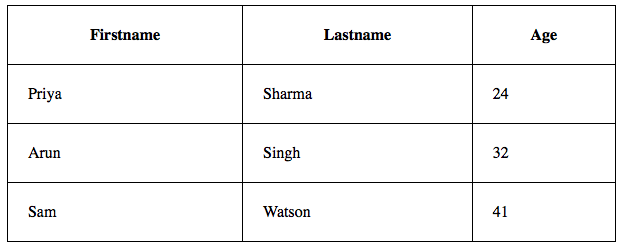Adding Left Align Headings in an HTML Table: By default, the table headings are bold and centered. To left-align the table headings, we must use the CSS text-align property.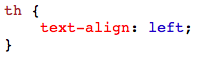Example 6: This example explains the text-align property where the text is aligned to the left.

## HTML

 `<``html``>`   `<``head``>` `    ``<``style``>` `    ``table,` `    ``th,` `    ``td {` `        ``border: 1px solid black;` `        ``border-collapse: collapse;` `    ``}` `    `  `    ``th,` `    ``td {` `        ``padding: 20px;` `    ``}` `    `  `    ``th {` `        ``text-align: left;` `    ``}` `    ``` ``   `<``body``>` `    ``<``table` `style``=``"width:100%"``>` `        ``<``tr``>` `            ``<``th``>Firstname` `            ``<``th``>Lastname` `            ``<``th``>Age` `        ``` `        ``<``tr``>` `            ``<``td``>Priya` `            ``<``td``>Sharma` `            ``<``td``>24` `        ``` `        ``<``tr``>` `            ``<``td``>Arun` `            ``<``td``>Singh` `            ``<``td``>32` `        ``` `        ``<``tr``>` `            ``<``td``>Sam` `            ``<``td``>Watson` `            ``<``td``>41` `        ``` `    ``` ``   ``

Output: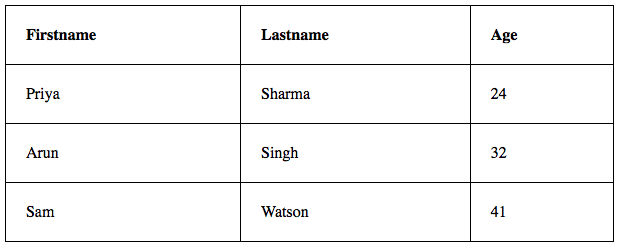text-align Property

Adding Border Spacing in an HTML Table: Border spacing specifies the space between the cells. To set the border-spacing for a table, we must use the CSS border-spacing property.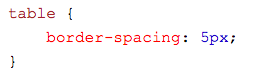Example 7: This example explains the border space property to make the space between the Table cells.

## HTML

 `<``html``>`   `<``head``>` `    ``<``style``>` `    ``table,` `    ``th,` `    ``td {` `        ``border: 1px solid black;` `    ``}` `    `  `    ``table {` `        ``border-spacing: 5px;` `    ``}` `    ``` ``   `<``body``>` `    ``<``table` `style``=``"width:100%"``>` `        ``<``tr``>` `            ``<``th``>Firstname` `            ``<``th``>Lastname` `            ``<``th``>Age` `        ``` `        ``<``tr``>` `            ``<``td``>Priya` `            ``<``td``>Sharma` `            ``<``td``>24` `        ``` `        ``<``tr``>` `            ``<``td``>Arun` `            ``<``td``>Singh` `            ``<``td``>32` `        ``` `        ``<``tr``>` `            ``<``td``>Sam` `            ``<``td``>Watson` `            ``<``td``>41` `        ``` `    ``` ``   ``

Output: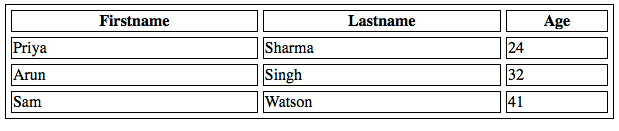Border Spacing Property

Adding Cells that Span Many Columns in HTML Tables: To make a cell span more than one column, we must use the colspan attribute.

Example 8: This example describes the use of the colspan attribute in HTML.

## HTML

 `` `<``html``>`   `<``head``>` `    ``<``style``>` `    ``table,` `    ``th,` `    ``td {` `        ``border: 1px solid black;` `        ``border-collapse: collapse;` `    ``}` `    `  `    ``th,` `    ``td {` `        ``padding: 5px;` `        ``text-align: left;` `    ``}` `    ``` ``   `<``body``>` `    ``<``h2``>Cell that spans two columns:` `    ``<``table` `style``=``"width:100%"``>` `        ``<``tr``>` `            ``<``th``>Name` `            ``<``th` `colspan``=``"2"``>Telephone` `        ``` `        ``<``tr``>` `            ``<``td``>Vikas Rawat` `            ``<``td``>9125577854` `            ``<``td``>8565557785` `        ``` `    ``` ``   ``

Output: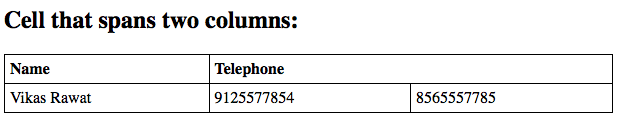colspan attribute

Adding Cells that span many rows in HTML Tables: To make a cell span more than one row, we must use the rowspan attribute.

Example 9: This example describes the use of the rowspan attribute in HTML.

## HTML

 `` `<``html``>`   `<``head``>` `    ``<``style``>` `    ``table,` `    ``th,` `    ``td {` `        ``border: 1px solid black;` `        ``border-collapse: collapse;` `    ``}` `    `  `    ``th,` `    ``td {` `        ``padding: 5px;` `        ``text-align: left;` `    ``}` `    ``` ``   `<``body``>` `    ``<``h2``>Cell that spans two rows:` `    ``<``table` `style``=``"width:100%"``>` `        ``<``tr``>` `            ``<``th``>Name:` `            ``<``td``>Vikas Rawat` `        ``` `        ``<``tr``>` `            ``<``th` `rowspan``=``"2"``>Telephone:` `            ``<``td``>9125577854` `        ``` `        ``<``tr``>` `            ``<``td``>8565557785` `        ``` `    ``` ``   ``

Output: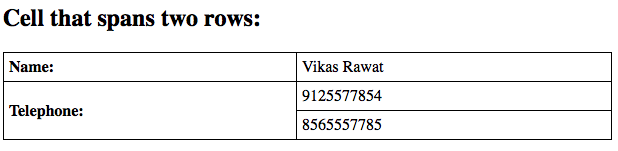Use of rowspan attribute

Adding a Caption in an HTML Table: To add a caption to a table, we must use the “caption” tag.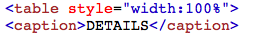Example 10: This example describes the HTML Table caption by specifying the CSS properties for setting its width.

## HTML

 `<``html``>`   `<``head``>` `    ``<``style``>` `    ``table,` `    ``th,` `    ``td {` `        ``border: 1px solid black;` `        ``border-collapse: collapse;` `    ``}` `    `  `    ``th,` `    ``td {` `        ``padding: 20px;` `    ``}` `    `  `    ``th {` `        ``text-align: left;` `    ``}` `    ``` ``   `<``body``>` `    ``<``table` `style``=``"width:100%"``>` `        ``<``caption``>DETAILS` `        ``<``tr``>` `            ``<``th``>Firstname` `            ``<``th``>Lastname` `            ``<``th``>Age` `        ``` `        ``<``tr``>` `            ``<``td``>Priya` `            ``<``td``>Sharma` `            ``<``td``>24` `        ``` `        ``<``tr``>` `            ``<``td``>Arun` `            ``<``td``>Singh` `            ``<``td``>32` `        ``` `        ``<``tr``>` `            ``<``td``>Sam` `            ``<``td``>Watson` `            ``<``td``>41` `        ``` `    ``` ``   ``

Output: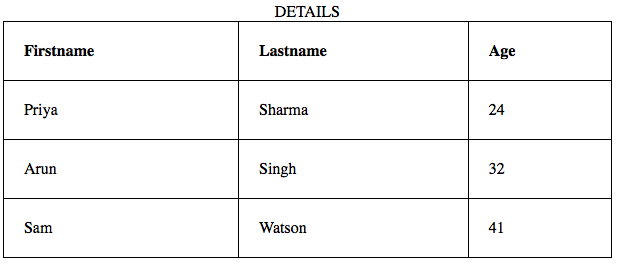Adding the caption using the <caption> tag

Adding a Background Colour to the Table: A color can be added as a background in an HTML table using the “background-color” option.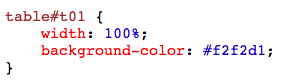Example 11: This example describes the addition of the Table background color in HTML.

## HTML

 `` `<``html``>`   `<``head``>` `    ``<``style``>` `    ``table,` `    ``th,` `    ``td {` `        ``border: 1px solid black;` `        ``border-collapse: collapse;` `    ``}` `    `  `    ``th,` `    ``td {` `        ``padding: 5px;` `        ``text-align: left;` `    ``}` `    `  `    ``table#t01 {` `        ``width: 100%;` `        ``background-color: #f2f2d1;` `    ``}` `    ``` ``   `<``body``>` `    ``<``table` `style``=``"width:100%"``>` `        ``<``tr``>` `            ``<``th``>Firstname` `            ``<``th``>Lastname` `            ``<``th``>Age` `        ``` `        ``<``tr``>` `            ``<``td``>Priya` `            ``<``td``>Sharma` `            ``<``td``>24` `        ``` `        ``<``tr``>` `            ``<``td``>Arun` `            ``<``td``>Singh` `            ``<``td``>32` `        ``` `        ``<``tr``>` `            ``<``td``>Sam` `            ``<``td``>Watson` `            ``<``td``>41` `        ``` `    ``` `    ``<``br` `/>` `    ``<``br` `/>` `    ``<``table` `id``=``"t01"``>` `        ``<``tr``>` `            ``<``th``>Firstname` `            ``<``th``>Lastname` `            ``<``th``>Age` `        ``` `        ``<``tr``>` `            ``<``td``>Priya` `            ``<``td``>Sharma` `            ``<``td``>24` `        ``` `        ``<``tr``>` `            ``<``td``>Arun` `            ``<``td``>Singh` `            ``<``td``>32` `        ``` `        ``<``tr``>` `            ``<``td``>Sam` `            ``<``td``>Watson` `            ``<``td``>41` `        ``` `    ``` ``   ``

Output: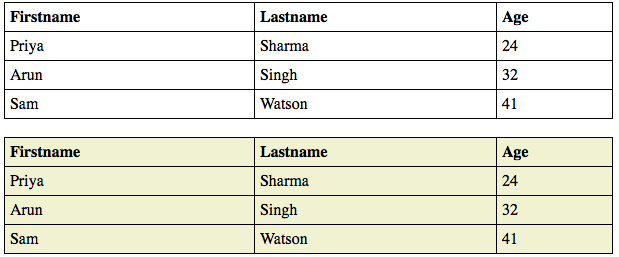Adding Table Background color using CSS properties

Creating Nested Tables: Nesting tables simply means making a Table inside another Table. Nesting tables can lead to complex tables layouts, which are visually interesting and have the potential of introducing errors.

Example 12: This example describes the Nested of HTML Table.

## HTML

 `` `<``html``>`   `<``body``>` `    ``<``table` `border``=``5` `bordercolor``=``black``>` `        ``<``tr``>` `            ``<``td``> First Column of Outer Table ` `            ``<``td``>` `                ``<``table` `border``=``5` `bordercolor``=``grey``>` `                    ``<``tr``>` `                        ``<``td``> First row of Inner Table ` `                    ``` `                    ``<``tr``>` `                        ``<``td``> Second row of Inner Table ` `                    ``` `                ``` `            ``` `        ``` `    ``` ``   ``

Output: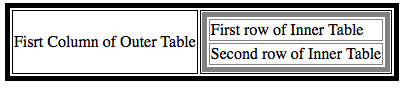Nested HTML Table

Supported Browsers:

• Google Chrome 1 and above
• Edge 12 and above
• Firefox 1 and above
• Internet Explorer
• Safari
• Opera

HTML is the foundation of webpages, is used for webpage development by structuring websites and web apps. You can learn HTML from the ground up by following this HTML Tutorial and HTML Examples.

Whether you're preparing for your first job interview or aiming to upskill in this ever-evolving tech landscape, GeeksforGeeks Courses are your key to success. We provide top-quality content at affordable prices, all geared towards accelerating your growth in a time-bound manner. Join the millions we've already empowered, and we're here to do the same for you. Don't miss out - check it out now!

Previous
Next